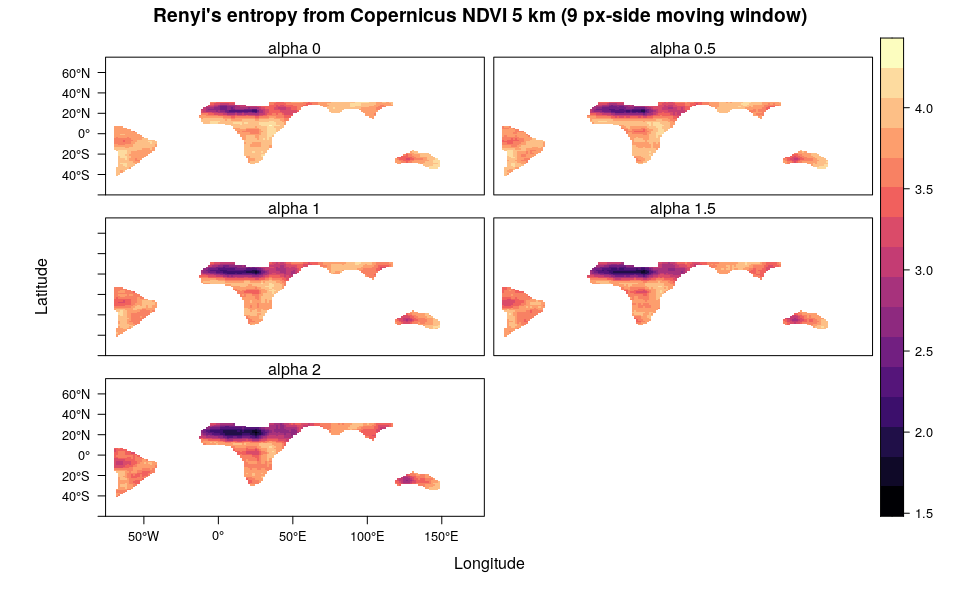# Rasterdiv: Derive indices of diversity from NDVI.

#### 2022-03-29

require(rasterdiv)
require(rasterVis)
require(RColorBrewer)

This vignette uses rasterdiv to build global series of indices of diversity based on Information Theory. The input dataset is the Copernicus Long-term (1999-2017) average Normalised Difference Vegetation Index for the 21st of June (copNDVI).

## Overview

A RasterLayer called copNDVI is loaded together with the package rasterdiv. copNDVI is a 8-bit raster, meaning that pixel values range from 0 to 255. You could stretch it to match a more familiar (-1,1) values range using raster::stretch(copNDVI,minv=-1,maxv=1) .

## Reclassify NDVI

Pixels with values 253, 254 and 255 (water) will be set as NA’s.

copNDVI <- raster::reclassify(copNDVI, cbind(252,255, NA), right=TRUE)

## Resample NDVI to a coarser resolution

To speed up the calculation, the RasterLayer will be “resampled” at a resolution 10 times coarser than original and cut on Africa.

#Resample using raster::aggregate and a linear factor of 20
copNDVIlr <- raster::aggregate(copNDVI, fact=20)
#Set float numbers as integers to further speed up the calculation
storage.mode(copNDVIlr[]) = "integer"

## Compare NDVI low and high resolution

levelplot(copNDVI,layout=c(0,1,1), main="NDVI 21st of June 1999-2017 - ~8km pixel resolution")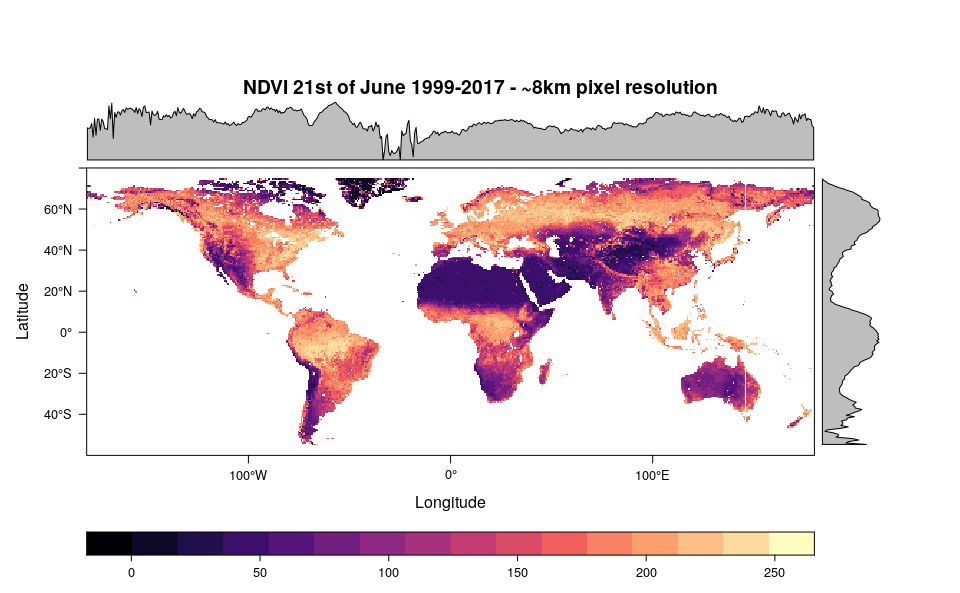levelplot(copNDVIlr,layout=c(0,1,1), main="NDVI 21st of June 1999-2017 - ~150km pixel resolution")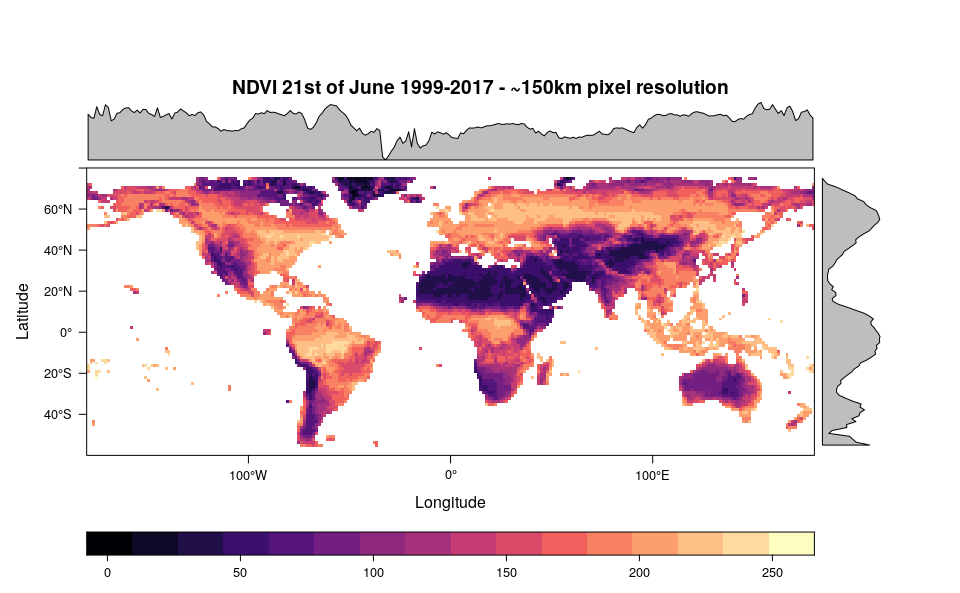## Compute Area based Rao’s Index

rasterdiv allows to derive area-based Rao’s index from input vector and raster layers.

RaoC <- paRao(x=copNDVIlr, area=world, field='CONTINENT', alpha=c(1,2))
#Plot area-based RAo's index
plot(RaoC, col=hcl(RaoC$alpha.1*10), main="Rao's index per continent alpha 1") text(RaoC, label=paste("Rao'Q =", round(RaoC$alpha.1,1)), col="black", family="Arial")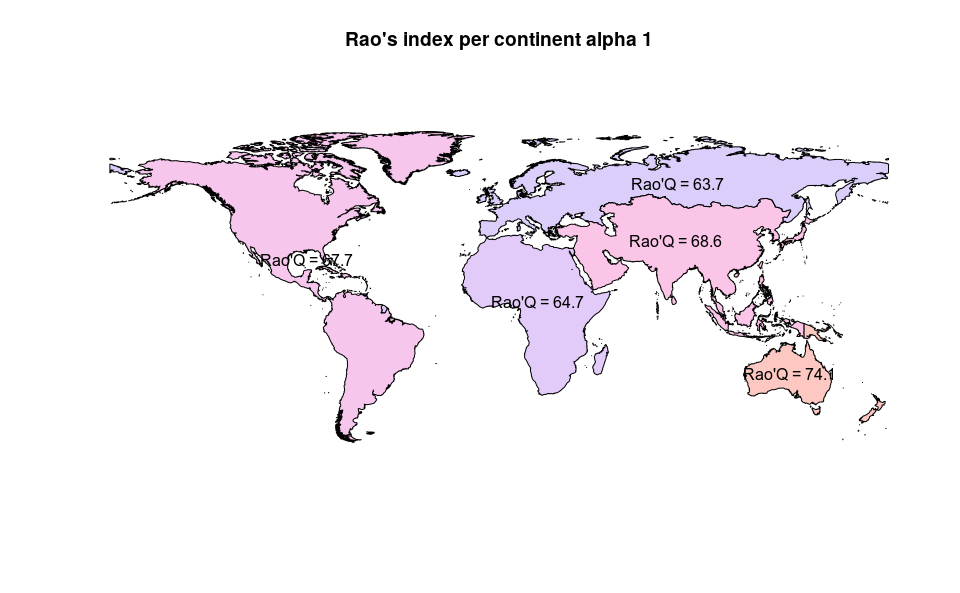## Compute all indexes in rasterdiv

rasterdiv allows the computation of 8 diversity indexes based on information theory. In the following section, all these indexes will be computed for copNDVIlr using a moving window of 81 pixels (9 px side). Alpha values for the Hill, Rényi and parametric Rao indexes will be set from 0 to 2 every 0.5. In addition, we will set na.tolerance=0.1, meaning that all moving windows with more than 10% of pixels equal NA will be set to NA.

#Shannon's Diversity
sha <- Shannon(copNDVIcont,window=9,na.tolerance=0.1,np=1)

#Pielou's Evenness
pie <- Pielou(copNDVIcont,window=9,na.tolerance=0.1,np=1)

#Berger-Parker's Index
ber <- BergerParker(copNDVIcont,window=9,na.tolerance=0.1,np=1)

#Parametric Rao's quadratic entropy with alpha ranging from 1 to 5
prao <- paRao(copNDVIcont,window=9,alpha=1:5,na.tolerance=0.1,dist_m="euclidean",np=1)

#Cumulative Residual Entropy
cre <- CRE(copNDVIcont,window=9,na.tolerance=0.1,np=1)

#Hill's numbers
hil <- Hill(copNDVIcont,window=9,alpha=seq(0,2,0.5),na.tolerance=0.1,np=1)

#Rényi's Index
ren <- Renyi(copNDVIcont,window=9,alpha=seq(0,2,0.5),na.tolerance=0.1,np=1)

## Visualise RasterLayers

#Shannon's Diversity
levelplot(sha,main="Shannon's entropy from Copernicus NDVI 5 km (9 px-side moving window)",as.table = T,layout=c(0,1,1), ylim=c(-60,75), margin = list(draw = TRUE))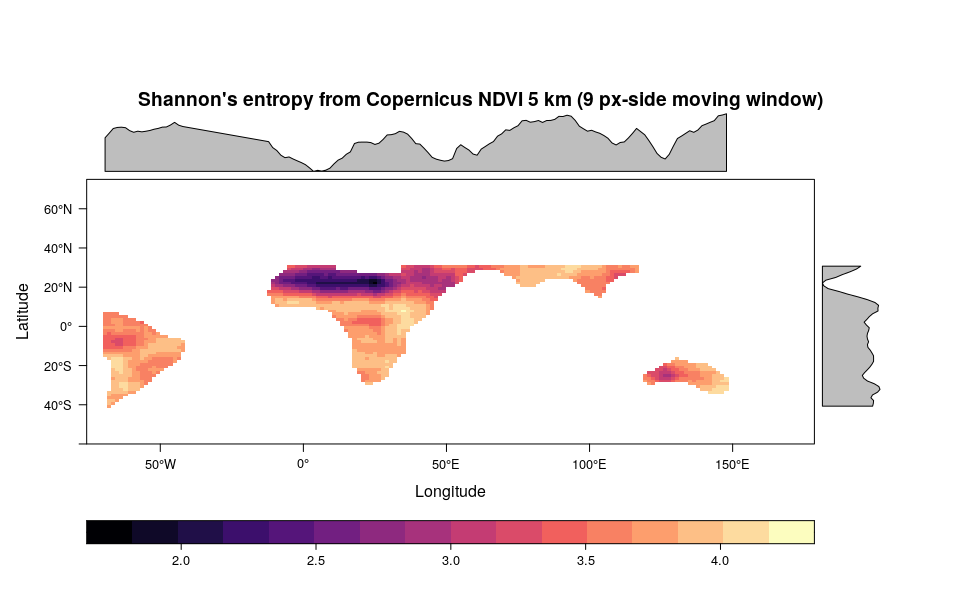#Pielou's Evenness
levelplot(pie,main="Pielou's evenness from Copernicus NDVI 5 km (9 px-side moving window)",as.table = T,layout=c(0,1,1), ylim=c(-60,75), margin = list(draw = TRUE))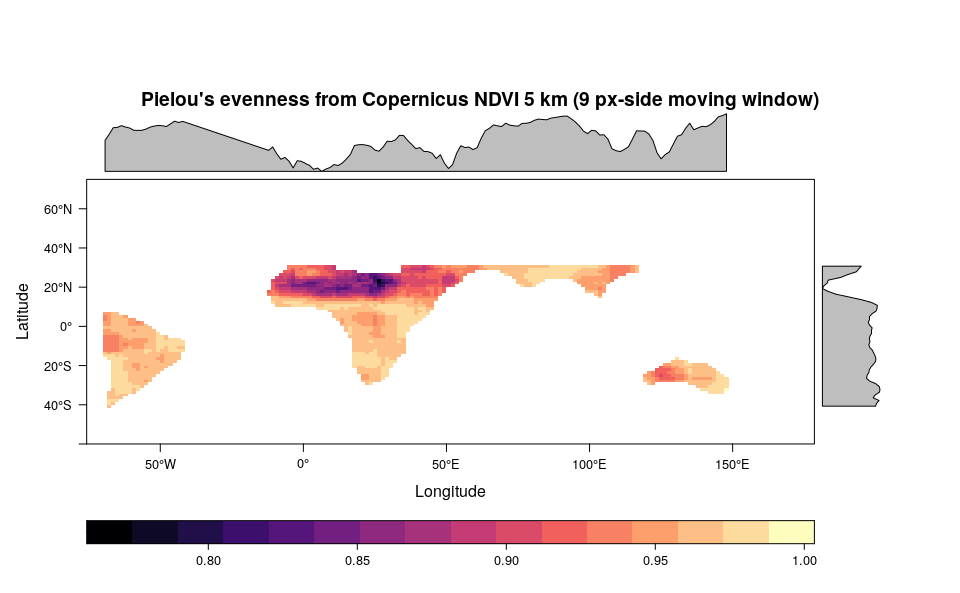#Berger-Parker' Index
levelplot(ber,main="Berger-Parker's index from Copernicus NDVI 5 km (9 px-side moving window)",as.table = T,layout=c(0,1,1), ylim=c(-60,75), margin = list(draw = TRUE))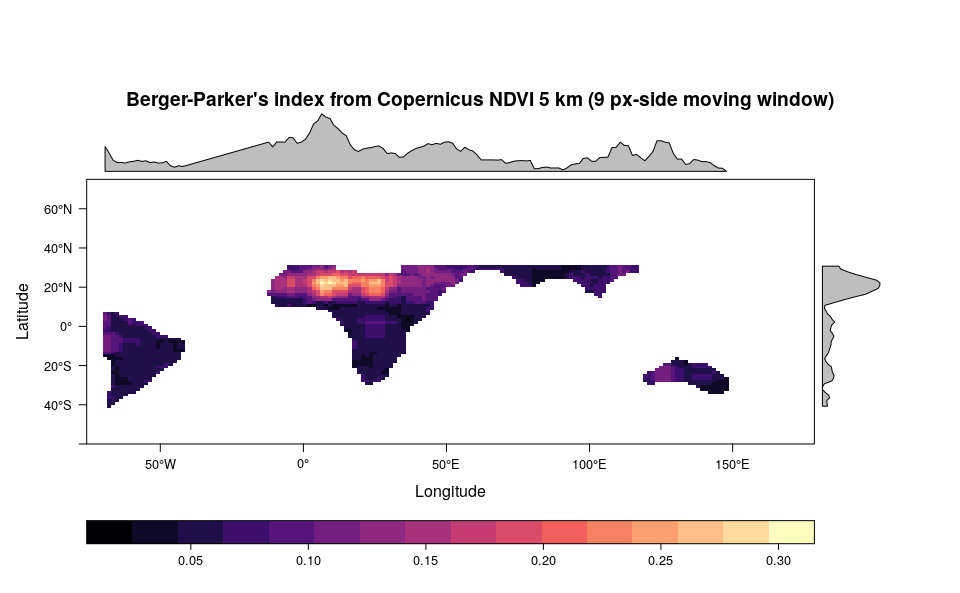#Parametric Rao's quadratic Entropy
levelplot(stack(prao[]),main="Parametric Rao's quadratic entropy from Copernicus NDVI 5 km (9 px-side moving window)",as.table = T,layout=c(0,5,1), ylim=c(-60,75), margin = list(draw = TRUE))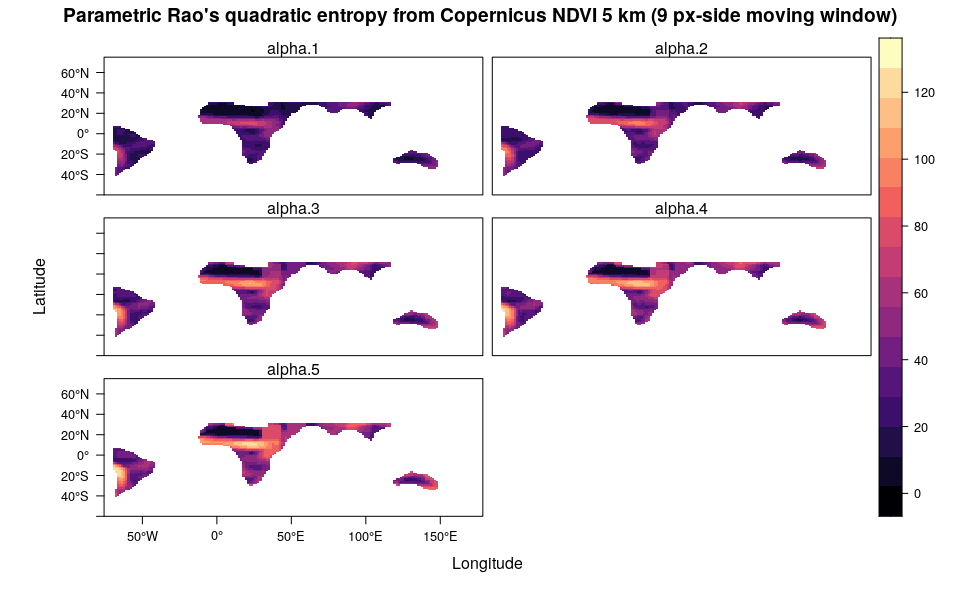#Cumulative Residual Entropy
levelplot(cre,main="Cumulative Residual Entropy from Copernicus NDVI 5 km (9 px-side moving window)",as.table = T,layout=c(0,1,1), ylim=c(-60,75), margin = list(draw = TRUE))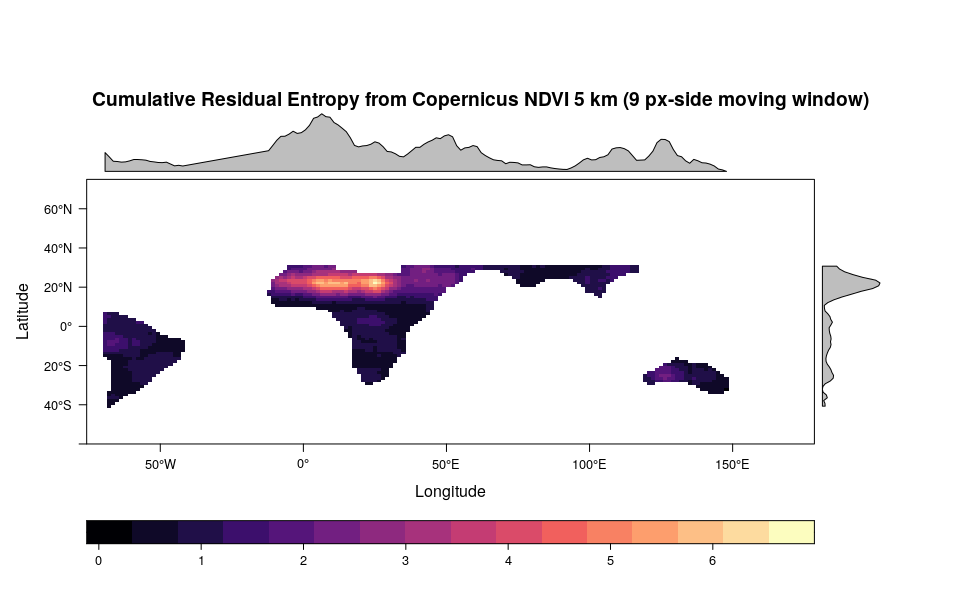#Hill's numbers (alpha=0, 0.5, 1, 1.5 and 2)
levelplot(stack(hil),main="Hill's numbers from Copernicus NDVI 5 km (9 px-side moving window)",as.table = T,layout=c(0,5,1), ylim=c(-60,75))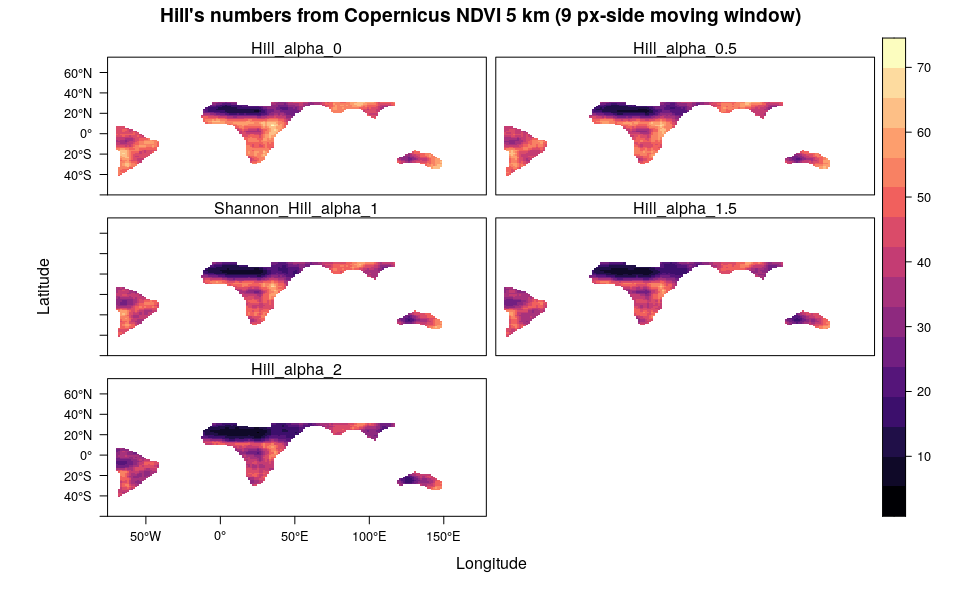#Renyi' Index (alpha=0, 1, 1.5 and 2)
levelplot(stack(ren),main="Renyi's entropy from Copernicus NDVI 5 km (9 px-side moving window)",as.table = T,layout=c(0,5,1),names.attr=paste("alpha",seq(0,2,0.5),sep=" "), ylim=c(-60,75), margin = list(draw = FALSE))